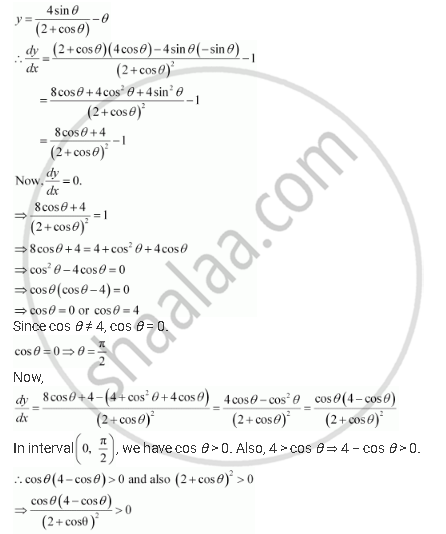Share

# Prove That Y = (4sin Theta)/(2 + Cos Theta) - Theta Is an Increasing Function Of θ In [0, Pi/2] - CBSE (Commerce) Class 12 - Mathematics

ConceptIncreasing and Decreasing Functions

#### Question

Prove that  y = (4sin theta)/(2 + cos theta) - theta is an increasing function of θ in [0, pi/2]

#### Solution

We have,=> dy/dx > 0

Therefore, y is strictly increasing in interval (0, pi/2)

Also, the given function is continuous at x = 0 and x = pi/2

Hence, y is increasing in interval  [0, pi/2]

Is there an error in this question or solution?

#### APPEARS IN

NCERT Solution for Mathematics Textbook for Class 12 (2018 to Current)
Chapter 6: Application of Derivatives
Q: 9 | Page no. 205

#### Video TutorialsVIEW ALL 

Solution Prove That Y = (4sin Theta)/(2 + Cos Theta) - Theta Is an Increasing Function Of θ In [0, Pi/2] Concept: Increasing and Decreasing Functions.
S